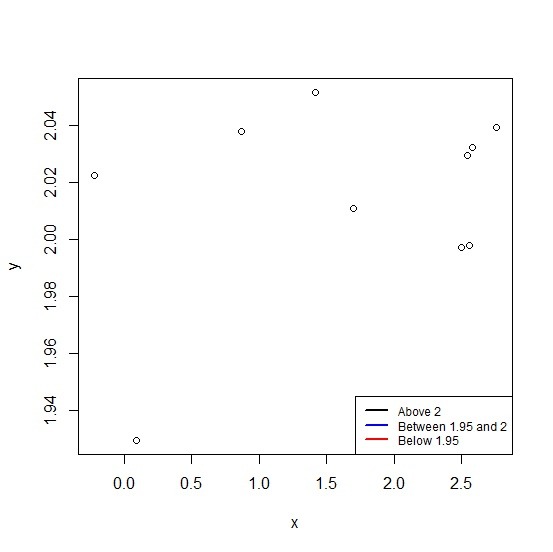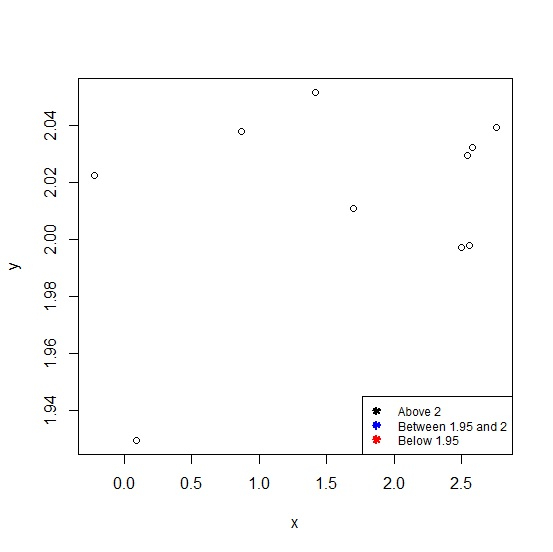# How to represent the legend in a plot created by using plot function with colored straight lines or stars in R?

A legend helps us to differentiate between the type of values or any another division of values in a data set. These legends can be represented in many ways and two of these ways are straight lines and stars. To represent the legend in a plot created by using plot function with colored straight lines or stars, we need to correct lty and pch arguments.

## Example

Consider the below vectors −

set.seed(199)
x<-rnorm(10,2,1)
y<-rnorm(10,2,0.04)

Creating the plot between x and y with colored lines legend values −

plot(x,y) legend("bottomright",legend=c("Above 2","Between 1.95 and 2","Below 1.95"), + lwd=2,cex=0.75,col=c("black","blue","red"),lty=c(1,1,1))

## OutputCreating the plot between x and y with colored stars legend values −

plot(x,y) legend("bottomright",legend=c("Above 2","Between 1.95 and 2","Below 1.95"), + lwd=2,cex=0.75,col=c("black","blue","red"),lty=c(NA,NA,NA),pch=c(8,8,8))

## Output